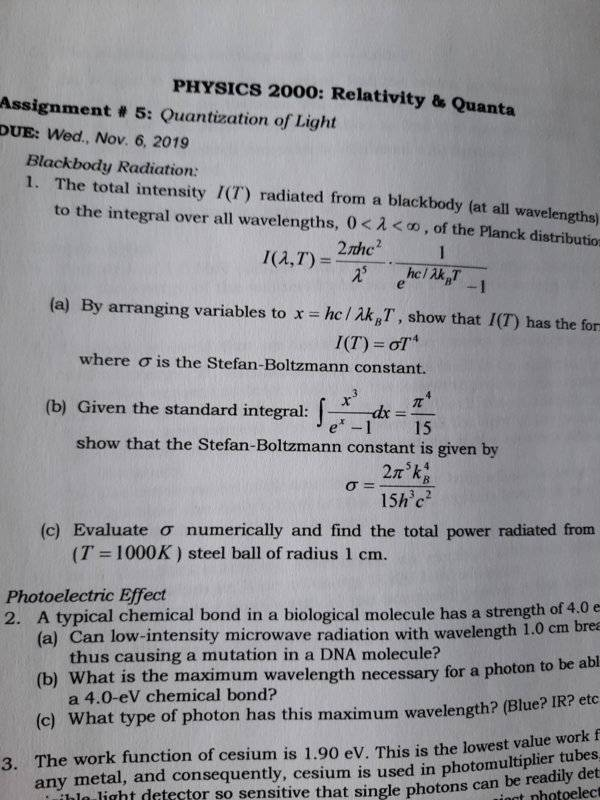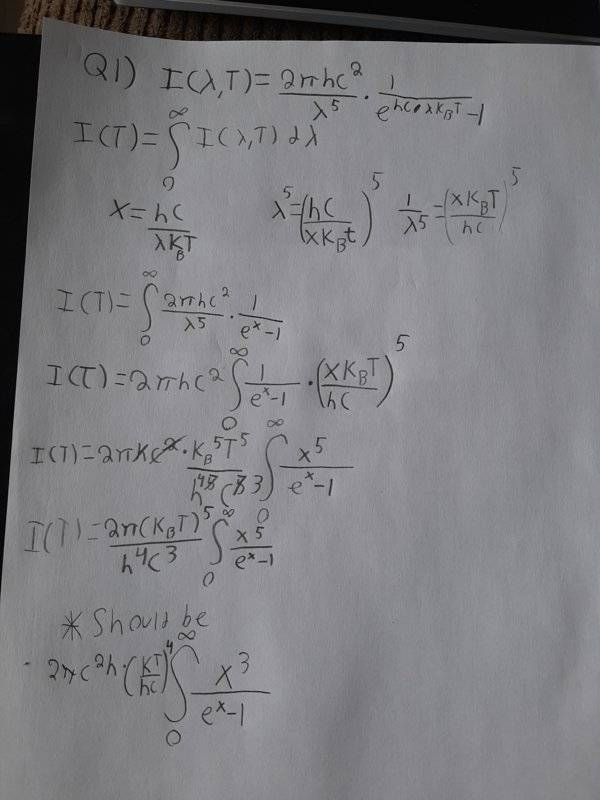# Black body radiation and the derivation of Stefan Boltzman

• dcarmichael

#### dcarmichael

Homework Statement
The total intensity i(t) radiated from a blackbody is given by the integral from 0 to infinity of all wavelengths of the Planck distribution.But I keep seem to be getting the wrong answer. Could someone point out where I'm going wrong
Relevant Equations
Let l=lambda I(l,T)=(2Pihc^2)/l^5 *1/(e^(hc/lkT)-1)What did you replace dλ with when converting to x as the variable of integration?

What did you replace dλ with when converting to x as the variable of integration?
I didnt indicate it but dλ is replaced with dx since x is new variable of integration

I didnt indicate it but dλ is replaced with dx since x is new variable of integration
##d\lambda \neq dx##

Note that you can write ##\lambda = \large \frac{a}{x}##, where ##a## is a constant. Taking the differential of both sides of this relation, you should get ##d\lambda = \boxed ?\, dx##. What goes inside the box?

##d\lambda \neq dx##

Note that you can write ##\lambda = \large \frac{a}{x}##, where ##a## is a constant. Taking the differential of both sides of this relation, you should get ##d\lambda = \boxed ?\, dx##. What goes inside the box?
dλ= -(a/x^2)dx

Ok. Go for it.